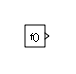# Function-Call Generator

Provide function-call events to control execution of a subsystem or model

• Library:
• Simulink / Ports & Subsystems

•## Description

The Function-Call Generator block provides function-call events that execute a function-call subsystem or function-call model at the rate that you specify with the Sample time parameter. To iteratively execute each function-call block multiple times at each time step, use the Number of Iterations parameter.

To execute multiple function-call subsystems or models in a specified order, use the Function-Call Generator block with a Function-Call Split block. For an example, see the Function-Call Split block documentation.

expand all

## Parameters

expand all

Specify the time interval between function call events to a subsystem or model containing a Trigger block with Trigger type set to `function-call`. If the actual calling rate for the subsystem or model differs from the time interval this parameter specifies, Simulink® displays an error.

#### Settings

`-1`

Inherit time interval from the model.

`Ts`

Scalar where `Ts` is the time interval.

`[Ts, To]`

Vector where `Ts` is the time interval and `To` is the initial time offset.

#### Programmatic Use

 Block Parameter: `sample_time` Type: character vector Values: `'-1'` | `'Ts'`| `'[Ts, To]'` Default: `'-1'`

The value of this parameter can be a vector where each element of the vector specifies a number of times to execute a function-call subsystem. The total number of times that a function-call subsystem executes per time step is equal to the sum of vector element values.

Suppose that you specify the number of iterations to be ```[2 2]``` and connect the output of this block to the control port of a function-call subsystem. In this case, the function-call subsystem executes four times at each time step.

#### Settings

`1`

Provide function-call once during each time step.

integer

Signed or unsigned integer number. Provide the specified number of function calls at each time step.

#### Programmatic Use

 Block Parameter: `numberOfIterations` Type: character vector Values: `'1'` | `''` Default: `'1'`

## Block Characteristics

 Data Types Direct Feedthrough `no` Multidimensional Signals `no` Variable-Size Signals `no` Zero-Crossing Detection `no`